Next: 5.2.3 Recall Up: 5.2.2 Cylindrical potential Previous: 5.2.2 Cylindrical potential

### 5.2.2.1 Examples

We study two extreme cases as examples for potential fields. Let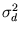be the maximal variance of the distribution, and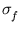be the size of the smallest structure of interest within the distribution. We use a Gaussian kernel, k(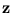,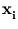) = exp(- ||-||2/(2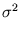)).

First case,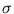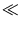: the kernel function k(,) is almost everywhere approximately zero, apart from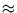. Therefore, the spherical potential (5.3) is almost everywhere one, and the projections on the principal components (5.6) are almost everywhere zero. Thus, the potential according to (5.8) is almost everywhere one.

Second case,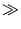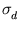: the kernel function k(,) is approximately one. If this value is put into (5.3), (5.6), and (5.8) the resulting potential value is zero (for all points).

Both cases do not give desirable potential fields. Therefore, the value ofshould be chosen from the interval [,].

Heiko Hoffmann
2005-03-22